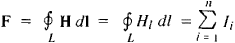# Magnetomotive Force

(redirected from magnetomotive forces)
Also found in: Dictionary, Thesaurus.

## magnetomotive force

[mag¦nēd·ō′mōd·iv ′fōrs]
(electromagnetism)
The work that would be required to carry a magnetic pole of unit strength once around a magnetic circuit. Abbreviated mmf.
McGraw-Hill Dictionary of Scientific & Technical Terms, 6E, Copyright © 2003 by The McGraw-Hill Companies, Inc.
The following article is from The Great Soviet Encyclopedia (1979). It might be outdated or ideologically biased.

## Magnetomotive Force

a quantity that characterizes the magnetic action of an electric current. The magnetomotive force is introduced in calculations of magnetic circuits by analogy with the electromotive force in electric circuits.

The magnetomotive force F is equal to the circulation of the vector of magnetic field intensity H through a closed circuit L that encompasses the electric currents generated by the magnetic field:(in the International System of Units). Here HI is the projection of H onto the direction of the element of the integration circuit d I and n is the number of conductors (coils) carrying a current Ii that are encompassed by the circuit. The unit of magnetomotive force is the ampere (or ampere-turn) in the International System of Units and the gilbert in the cgs system of units (symmetrical); 1 A = (4π/10) Gb ~ 1.2566 Gb.

References in periodicals archive ?
Analysis of the considered short pitch coil winding indicates, that during one pole pitch the relative magnetomotive force [F.sub.1]([phi]) has the shape, shown in Fig.
Space harmonic spectrum of each winding magnetomotive force can be obtained on the base of Fourier series .
where [A.sub.1] = 1/3 and [A.sub.2] = 2/3 are amplitudes of relative magnetomotive force components.
2(a), the conditional instantaneous changes in magnitude of magnetomotive force below the slots of magnetic circuit in the instant of time t = 0 are determined (Table III).
According to results from Table III, the space distribution of instantaneous rotating magnetomotive force in a defined moment of time was created (Fig.
For the analysed six-phase chain winding, the harmonic analysis equations of rotating magnetomotive force (Fig.
1, a, the conditional changes of magnetomotive force in the slots of magnetic circuit are determined (Table 3).
According to the results in Table 3 the spatial distribution of instantaneous rotating magnetomotive force in the set moment of time (t = 0) is determined (Fig 1, b).
here [F.sub.2v']--conditional amplitude value of the rotating magnetomotive force of the v'-th harmonics of the two-layer tree-phase fractional winding with q = 3/2; [F.sub.1v']--conditional amplitude value of the rotating magnetomotive force of the v'-th harmonics of the concentrated three-phase winding.
1, b, the amplitude value of the rotating magnetomotive force of the v-th harmonics [F.sub.2v] is calculated according to these analytical expressions :
To achieve the distribution of rotating magnetomotive force in the air gap of electric machines and the form of the curve of electromotive force induced in the windings as close as possible to sinusoidal, some certain measures are taken: the span of the windings is shortened (y < t), windings are distributed (q > 1), skewed slots are made in the magnetic circuit [1 - 2].
Functions of instantaneous periodic rotating magnetomotive force of concentrated three-phase winding are square-shaped.

Site: Follow: Share:
Open / Close